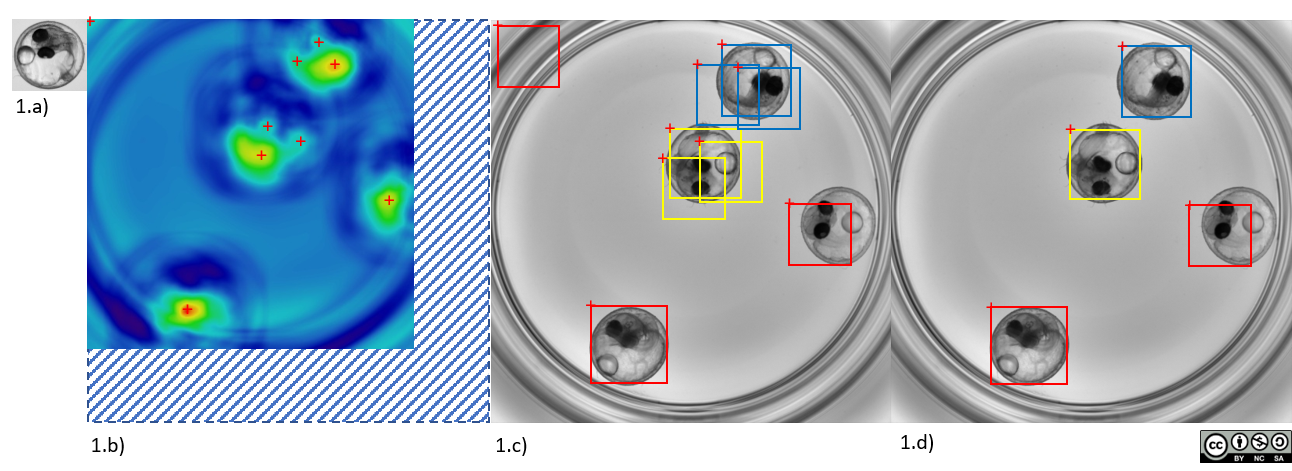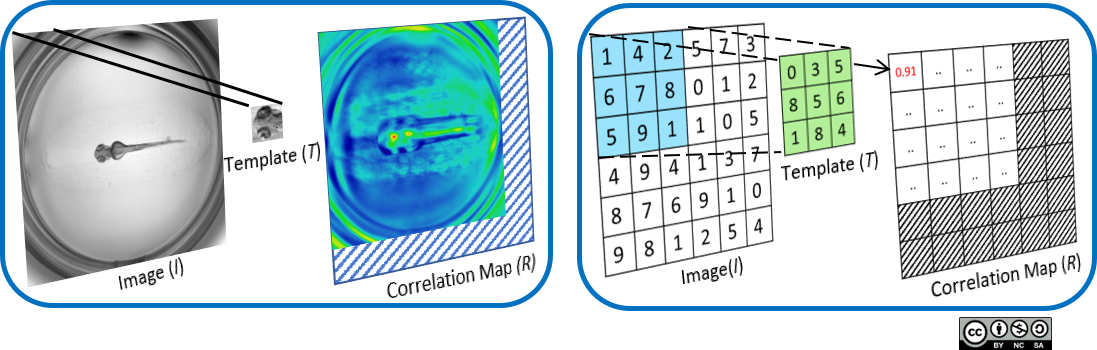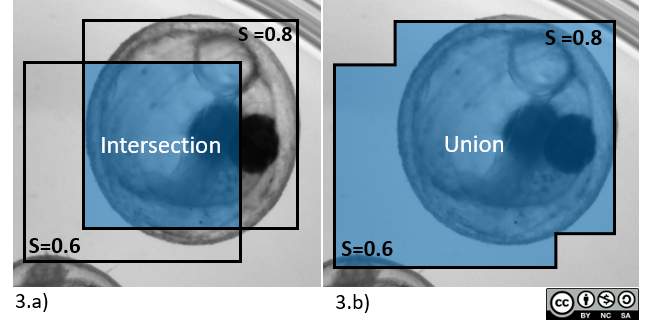View on GitHub

# Multi-Template Matching for object-detection

## Overview1.a) Template and 1.c), 1.d) searched image
1.b) Correlation map with local extrema overlapped as red crosses
1.c) Corresponding predicted locations as bounding boxes of dimensions identical to the template used for the search
1.d) Final yielded detections after Non-Maxima Suppression to return the N_object=4 best detections without overlap

## 1) Correlation map

The algorithm uses the template image as a sliding window translated over the image, and at each position of the template computes a similarity score between the template and the image patch.
This results in a correlation map, for which the pixel value at position (x,y) is proportional to the similarity between the template and image patch, at this (x,y) position in the image.

Different formulas can be used to compute the similarity score. See section Parameters.
With a correlation score, a high probability to find the object corresponds to a high score value.
With a difference score, a high probability correspond to a low score (low difference between the pixel values).

The correlation map is computed for each image with every template provided.## 2) Extrema detection

The computation of the correlation map is followed by extrema detection to list the possible locations of the template in the image. Each extrema corresponds to the possible location of a bounding box of dimensions identical to the template as illustrated below in Figure 2.c).

If a single object is expected in the image, the algorithm simply detects the global extremum of the correlation map and terminates there.
If more objects are expected, the algorithm performs extrema detection on the correlation map followed by Non-Maxima Suppression to return the best N non-overlapping detections.

The extrema detection can list a large number of extrema in the first place. Usually a threshold on the score is then used to limit this number (i.e. returning local extrema with a score above/below the threshold).
To prevent overlapping detection of the same object, Non-Maxima Supression is performed after extrema detection.

## 3) Non-Maxima Supression (NMS)

This additional step removes bounding boxes which overlap too much with bounding boxes of higher score.
The degree of overlap is measured by the ratio of the Intersection (3.a) over Union (3.b) (IoU) of the pair of bounding boxes.
A user-defined threshold on the IoU is used as the maximal value of overlap allowed between bounding boxes, such that if a bounding box overlaps above the IoU threshold with a bounding box of higher score, then this lower score bounding box is deleted. This effectively removes overlapping detection of the same object, while still allowing the detections of close objects for which the bounding boxes might slightly overlap.
If multiple templates are provided, the NMS is performed on the full set of extrema from the different correlation maps.## 4) Resulting detections

The NMS does not compute the overlap between all possible overlapping bounding boxes. It actually processes from the highest score bounding boxes to the lowest score ones, until it collects N bounding boxes (with N the number of expected objects in each image) or until there are no more bounding boxes. In this latter case, the number of returned bounding boxes can be below N.